## A Black-Scholes Option Calculator as a Table-Valued-Function

May 20

Written by: Charles Flock
5/20/2009 8:51 PMIn this blog I will demonstrate how to build a simple Black-Scholes options calculator by creating a table-valued function and using the XLeratorDB/statistics functions module.
One of the great financial engineering innovations of the twentieth-century was the development of formulae to evaluate options. The grandfather of all of these is the eponymous Black-Scholes options pricing model. If you do a search on Black-Scholes you will discover many entries on tools, and calculators, and add-ins for EXCEL, but I was unable to find anything for SQL Server. In this blog, I will demonstrate how to build a Black-Scholes calculation capability in SQL Server. You don’t need to know anything about the math behind this to follow along. And, if you are familiar with the math, there are some are probably things that can be added to make the calculation more suited to your needs. This is the beauty of creating the function in SQL Server; it will be completely under your control.

If, like me, you go to Wikipedia and look up the formula, you will discover that it’s this: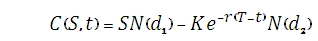Where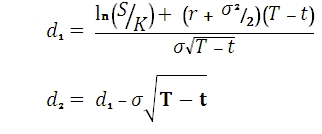And
S is the price of the stock,
C(s, t) is the price of a European call,
K is the strike price of the option
r is the annualized risk free interest rate, continuously compounded
s is the volatility of the stock
t is a time in years where now = 0 and expiry = T
N(x) is the standard normal cumulative distribution function

Of course, this formula only gives you the price for a call option. This price of the put option is calculated from the price of the call option.

It will turn out that we can actually calculate the price of the put option from the price of the call option.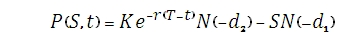Before we commence building our function, a couple of caveats. I am not an options-pricing expert, so this implementation could have mistakes. And, just to keep things simple, I have ignored dividends, which are usually included in the calculation. However, we also be including some other measurements of options performance, generally called the ‘Greeks’, and I am trying to not let things get too out of hand. The ‘greeks’ are named delta, vega, theta, rho, lambda, and gamma.

First, let’s define the inputs into our function. These are the share price, the strike price of the option, the volatility, the time to expiration as percentage of the year, and the risk-free interest rate. Let’s call our function ‘bs1’ for Black-Scholes. We begin creating the function with:

CREATE FUNCTION [dbo].[bs1]
(
@Shareprice float,
@Strikeprice float,
@Volatility float,
@Time float,
@Rate float
)

Since we are creating a table-valued function (TVF) we also need to provide a variable name for the table and the names for the variables returned in the table. We add the following information:

RETURNS
@bs TABLE
(
d1 float NOT NULL,
d2 float NOT NULL,
Value_Call float NOT NULL,
Value_Put float NOT NULL,
Delta_call float NOT NULL,
Delta_put float NOT NULL,
Vega float NOT NULL,
theta_call float NOT NULL,
theta_put float NOT NULL,
Rho_call float NOT NULL,
Rho_put float NOT NULL,
lambda_call float NOT NULL,
lambda_put float NOT NULL,
Gamma float NOT NULL
)

Now we will declare the variables to be used in the calculations.

AS
BEGIN
DECLARE @d1 as float,
@d2 as float,
@Val_Call as float,
@Val_Put as float,
@Delta_call as float,
@Delta_put as float,
@phi_d1 as float,
@Vega as float,
@Theta_call as float,
@Theta_put as float,
@Rho_call as float,
@Rho_put as float,
@lambda_call as float,
@lambda_put as float,
@Gamma as float

And now we are going to add the calculations.

First we need calculate the variable called @d1.

set @d1 =(Log(@shareprice / @strikeprice) +(@rate + POWER(@Volatility,2) / 2.0) * @time) /(@Volatility * Sqrt(@time))
Next we calculate the variable called @d2.

set @d2 = @d1 -(@Volatility * Sqrt(@Time))
And, now we are ready to calculate the value of the call option, using the XLeratorDB NORMSDST function.

set @Val_call = @Shareprice * wct.NORMSDIST(@d1) - @Strikeprice * EXP(-@rate*@time) * wct.NORMSDIST(@d2)
Having calculated the price of the call option, we can calculate the price of the put opion.

set @Val_put =(@Strikeprice * EXP(-@rate*@time) * wct.NORMSDIST(-@d2)) -(@Shareprice * wct.NORMSDIST(-@d1))
We can begin calculating the Greeks. The first of these is delta, which measures the rate of change of option value with respect to changes in the underlying asset’s price.

set @Delta_Call = wct.NORMSDIST(@d1)
set @Delta_Put = -wct.NORMSDIST(-@d1)
Next, we will calculate vega, and another variable that I have called @phi_d1 which is used in several of the Greeks calculations.

set @Phi_d1 =(1 / Sqrt(2 * PI())) *(exp(-0.5 * power(@d1, 2)))
set @Vega = @Shareprice * Sqrt(@Time) * @Phi_d1 * .01
Theta measures sensitivity of the value of an option to the passage of time.

set @Theta_call = -(@Shareprice * @Volatility * @Phi_d1 /(2 * Sqrt(@Time))) -(@Rate *(@Strikeprice * EXP(-@rate*@time)) * wct.NORMSDIST(@d2))
set @Theta_call = @Theta_call / 365
set @Theta_put = -(@Shareprice * @Volatility * @Phi_d1 /(2 * Sqrt(@Time))) +(@Rate *(@Strikeprice * EXP(-@rate*@time)) * wct.NORMSDIST(-@d2))
set @Theta_put = @Theta_put / 365
Rho measures sensitivity to the applicable interest rate.

set @Rho_Call = @Time *(@Strikeprice * EXP(-@rate*@time)) * wct.NORMSDIST(@d2) * .01
set @Rho_put = -@Time *(@Strikeprice* EXP(-@rate*@time)) * wct.NORMSDIST(-@d2) * .01
Lambda measures the percentage change in option value per change in the underlying price.

set @Lambda_Call = @Delta_Call * @Shareprice / @Val_call
set @Lambda_Put = @Delta_Put * @Shareprice / @Val_put
Gamma measures the rate of change in the delta with respect to changes in the underlying price.

set @gamma = @Phi_d1 / (@Shareprice * @Volatility * Sqrt(@Time))

Finally, we insert all the calculated values into the table variable.

INSERT @bs
SELECT @d1, @d2, @Val_call, @Val_put, @delta_call, @Delta_put, @Vega, @Theta_call, @Theta_put, @Rho_Call, @Rho_put, @lambda_Call, @Lambda_put, @Gamma
RETURN
END

Create the function, and then we are ready to look at how it works in practice. Let’s look at a call option with a strike price of 24.50, settling on 18 May 2009 with an expiry date of 12 June 2009. The price of the underlying security is 25.00, the volatility is 30% and the Risk Free Rate is 3.50%. Since we built our model with the time variable as a fraction of the year, we will need to convert it as we pass it in, using the XLeratorDB YEARFRAC function.

select Value_call
from bs1(25,24.50,.30,wct.YEARFRAC('05/18/2009','06/12/2009',3), .035)
This produces the following result.

Value_call
----------------------
1.08527523955875)

If we also wanted to include the value of the put:
select Value_call
,Value_put
from bs1(25,24.50,.30,wct.YEARFRAC('05/18/2009','06/12/2009',3), .035)
This produces the following result.

Value_call              Value_put
---------------------- ----------------------
1.08527523955875      0.526612705621268

In this SELECT where are choosing several of the Greeks values with the call value being in the left 6 columns and the put values being in the right 6 columns. I have also used the XLeratorDB TEXT function to format the numbers and round them to 4 decimal places, simply to make the output a little more readable.

select left(wct.TEXT(Value_call, '#,#.0000'), 8) as [Call Price]
,left(wct.TEXT(Delta_call, '#,#.0000'), 8) as [Call Delta]
,left(wct.TEXT(Gamma, '#,#.0000'), 8) as [Call Gamma]
,left(wct.TEXT(Vega, '#,#.0000'), 8) as [Call Vega]
,left(wct.TEXT(Theta_call, '#,#.0000'), 8) as [Call Theta]
,left(wct.TEXT(Rho_call, '#,#.0000'), 8) as [Call Rho]
,left(wct.TEXT(Value_put, '#,#.0000'), 8) as [Put Price]
,left(wct.TEXT(Delta_put, '#,#.0000'), 8) as [Put Delta]
,left(wct.TEXT(Gamma, '#,#.0000'), 8) as [Put Gamma]
,left(wct.TEXT(Vega, '#,#.0000'), 8) as [Put Vega]
,left(wct.TEXT(Theta_put, '#,#.0000'), 8) as [Put Theta]
,left(wct.TEXT(Rho_put, '#,#.0000'), 8) as [Put Rho]
from bs1(25,24.50,.30,wct.YEARFRAC('05/18/2009','06/12/2009',1), .035)
This produces the following result.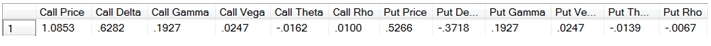Finally, we can calculate the option values and the Greeks for a range of strike prices by using a CTE.
;with opt as
(
select cast(22.50 as float) as strike_price
,left(wct.TEXT(Value_call, '#,#.0000'), 8) as [Call Price]
,left(wct.TEXT(Delta_call, '#,#.0000'), 8) as [Call Delta]
,left(wct.TEXT(Gamma, '#,#.0000'), 8) as [Call Gamma]
,left(wct.TEXT(Vega, '#,#.0000'), 8) as [Call Vega]
,left(wct.TEXT(Theta_call, '#,#.0000'), 8) as [Call Theta]
,left(wct.TEXT(Rho_call, '#,#.0000'), 8) as [Call Rho]
,left(wct.TEXT(Value_put, '#,#.0000'), 8) as [Put Price]
,left(wct.TEXT(Delta_put, '#,#.0000'), 8) as [Put Delta]
,left(wct.TEXT(Gamma, '#,#.0000'), 8) as [Put Gamma]
,left(wct.TEXT(Vega, '#,#.0000'), 8) as [Put Vega]
,left(wct.TEXT(Theta_put, '#,#.0000'), 8) as [Put Theta]
,left(wct.TEXT(Rho_put, '#,#.0000'), 8) as [Put Rho]
from bs1(25,22.50,.30,wct.YEARFRAC('05/18/2009','06/12/2009',1), .035)
union all
select strike_price + .50
,left(wct.TEXT(Value_call, '#,#.0000'), 8) as [Call Price]
,left(wct.TEXT(Delta_call, '#,#.0000'), 8) as [Call Delta]
,left(wct.TEXT(Gamma, '#,#.0000'), 8) as [Call Gamma]
,left(wct.TEXT(Vega, '#,#.0000'), 8) as [Call Vega]
,left(wct.TEXT(Theta_call, '#,#.0000'), 8) as [Call Theta]
,left(wct.TEXT(Rho_call, '#,#.0000'), 8) as [Call Rho]
,left(wct.TEXT(Value_put, '#,#.0000'), 8) as [Put Price]
,left(wct.TEXT(Delta_put, '#,#.0000'), 8) as [Put Delta]
,left(wct.TEXT(Gamma, '#,#.0000'), 8) as [Put Gamma]
,left(wct.TEXT(Vega, '#,#.0000'), 8) as [Put Vega]
,left(wct.TEXT(Theta_put, '#,#.0000'), 8) as [Put Theta]
,left(wct.TEXT(Rho_put, '#,#.0000'), 8) as [Put Rho]
from opt
CROSS APPLY bs1(25,strike_price + .50,.30,wct.YEARFRAC('05/18/2009','06/12/2009',1), .035)
where strike_price < 27.50

) select * from OPT

This produces the following result.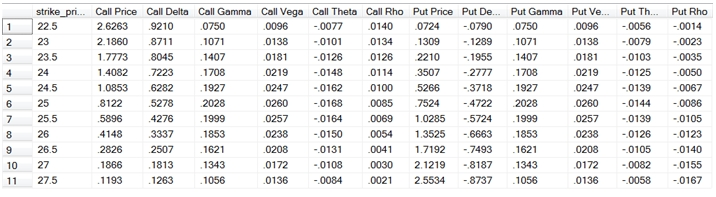As you can see, there are many different things that you can do using the XLeratorDB functions in conjunction with the user-defined function capability in SQL Server. Combining these functions with a scripting language like SQL provides you with many powerful solutions to problems that you had to pigeonhole into spreadsheet programs in the past.
I have also provided a function that will return all the option calculations in a neat, 3 column format, simply to demonstrate some of the creative solutions that are available to you. The script is provided below.
select *
from dbo.bs(25,24.50,.30,wct.YEARFRAC('05/18/2009','06/12/2009',3), .035)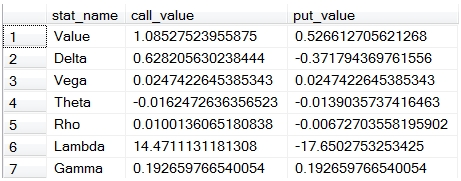CREATE FUNCTION [dbo].[bs]
(
-- Add the parameters for the function here
@Shareprice float,
@Strikeprice float,
@Volatility float,
@Time float,
@Rate float
)
RETURNS
@bs TABLE
(
-- Add the column definitions for the TABLE variable here
stat_name varchar(50),
call_value float,
put_value float
)
AS
BEGIN
-- Fill the table variable with the rows for your result set
DECLARE @d1 as float,
@d2 as float,
@Val_Call as float,
@Val_Put as float,
@Delta_call as float,
@Delta_put as float,
@phi_d1 as float,
@Vega as float,
@Theta_call as float,
@Theta_put as float,
@Rho_call as float,
@Rho_put as float,
@lambda_call as float,
@lambda_put as float,
@Gamma as float

set @d1 =(Log(@shareprice / @strikeprice) +(@rate + POWER(@Volatility,2) / 2.0) * @time) /(@Volatility * Sqrt(@time))
set @d2 = @d1 -(@Volatility * Sqrt(@Time))
set @Val_call = @Shareprice * wct.NORMSDIST(@d1) - @Strikeprice * EXP(-@rate*@time) * wct.NORMSDIST(@d2)
set @Val_put =(@Strikeprice * EXP(-@rate*@time) * wct.NORMSDIST(-@d2)) -(@Shareprice * wct.NORMSDIST(-@d1))
set @Delta_Call = wct.NORMSDIST(@d1)
set @Delta_Put = -wct.NORMSDIST(-@d1)
set @Phi_d1 =(1 / Sqrt(2 * PI())) *(exp(-0.5 * power(@d1, 2)))
set @Vega = @Shareprice * Sqrt(@Time) * @Phi_d1 * .01
set @Theta_call = -(@Shareprice * @Volatility * @Phi_d1 /(2 * Sqrt(@Time))) -(@Rate *(@Strikeprice * EXP(-@rate*@time)) * wct.NORMSDIST(@d2))
set @Theta_call = @Theta_call / 365
set @Theta_put = -(@Shareprice * @Volatility * @Phi_d1 /(2 * Sqrt(@Time))) +(@Rate *(@Strikeprice * EXP(-@rate*@time)) * wct.NORMSDIST(-@d2))
set @Theta_put = @Theta_put / 365
set @Rho_Call = @Time *(@Strikeprice * EXP(-@rate*@time)) * wct.NORMSDIST(@d2) * .01
set @Rho_put = -@Time *(@Strikeprice* EXP(-@rate*@time)) * wct.NORMSDIST(-@d2) * .01
set @Lambda_Call = @Delta_Call * @Shareprice / @Val_call
set @Lambda_Put = @Delta_Put * @Shareprice / @Val_put
set @gamma = @Phi_d1 /(@Shareprice * @Volatility * Sqrt(@Time))

INSERT into @bs VALUES ('Value', @Val_call, @Val_put)
INSERT into @bs VALUES ('Delta', @Delta_call, @Delta_put)
INSERT into @bs VALUES ('Vega', @Vega, @Vega)
INSERT into @bs VALUES ('Theta', @Theta_call, @Theta_put)
INSERT into @bs VALUES ('Rho', @Rho_call, @Rho_put)
INSERT into @bs VALUES ('Lambda', @lambda_call, @lambda_put)
INSERT into @bs VALUES ('Gamma', @Gamma, @Gamma)
RETURN
END

GO

CREATE FUNCTION [dbo].[bs1]
(
-- Add the parameters for the function here
@Shareprice float,
@Strikeprice float,
@Volatility float,
@Time float,
@Rate float
)
RETURNS
@bs TABLE
(
-- Add the column definitions for the TABLE variable here
d1 float NOT NULL,
d2 float NOT NULL,
Value_Call float NOT NULL,
Value_Put float NOT NULL,
Delta_call float NOT NULL,
Delta_put float NOT NULL,
Vega float NOT NULL,
theta_call float NOT NULL,
theta_put float NOT NULL,
Rho_call float NOT NULL,
Rho_put float NOT NULL,
lambda_call float NOT NULL,
lambda_put float NOT NULL,
Gamma float NOT NULL
)
AS
BEGIN
-- Fill the table variable with the rows for your result set
DECLARE @d1 as float,
@d2 as float,
@Val_Call as float,
@Val_Put as float,
@Delta_call as float,
@Delta_put as float,
@phi_d1 as float,
@Vega as float,
@Theta_call as float,
@Theta_put as float,
@Rho_call as float,
@Rho_put as float,
@lambda_call as float,
@lambda_put as float,
@Gamma as float

set @d1 =(Log(@shareprice / @strikeprice) +(@rate + POWER(@Volatility,2) / 2.0) * @time) /(@Volatility * Sqrt(@time))
set @d2 = @d1 -(@Volatility * Sqrt(@Time))
set @Val_call = @Shareprice * wct.NORMSDIST(@d1) - @Strikeprice * EXP(-@rate*@time) * wct.NORMSDIST(@d2)
set @Val_put =(@Strikeprice * EXP(-@rate*@time) * wct.NORMSDIST(-@d2)) -(@Shareprice * wct.NORMSDIST(-@d1))
set @Delta_Call = wct.NORMSDIST(@d1)
set @Delta_Put = -wct.NORMSDIST(-@d1)
set @Phi_d1 =(1 / Sqrt(2 * PI())) *(exp(-0.5 * power(@d1, 2)))
set @Vega = @Shareprice * Sqrt(@Time) * @Phi_d1 * .01
set @Theta_call = -(@Shareprice * @Volatility * @Phi_d1 /(2 * Sqrt(@Time))) -(@Rate *(@Strikeprice * EXP(-@rate*@time)) * wct.NORMSDIST(@d2))
set @Theta_call = @Theta_call / 365
set @Theta_put = -(@Shareprice * @Volatility * @Phi_d1 /(2 * Sqrt(@Time))) +(@Rate *(@Strikeprice * EXP(-@rate*@time)) * wct.NORMSDIST(-@d2))
set @Theta_put = @Theta_put / 365
set @Rho_Call = @Time *(@Strikeprice * EXP(-@rate*@time)) * wct.NORMSDIST(@d2) * .01
set @Rho_put = -@Time *(@Strikeprice* EXP(-@rate*@time)) * wct.NORMSDIST(-@d2) * .01
set @Lambda_Call = @Delta_Call * @Shareprice / @Val_call
set @Lambda_Put = @Delta_Put * @Shareprice / @Val_put
set @gamma = @Phi_d1 /(@Shareprice * @Volatility * Sqrt(@Time))

INSERT @bs
SELECT @d1, @d2, @Val_call, @Val_put, @delta_call, @Delta_put, @Vega, @Theta_call, @Theta_put, @Rho_Call, @Rho_put, @lambda_Call, @Lambda_put, @Gamma
RETURN
END

GO

Tags:
Categories:
Location: BlogsThe WestClinTech Blog

## Search Blogs

 KeywordsPhrase

## Blog Archives

Archive
 < September 2023 >
SunMonTueWedThuFriSat
272829303112
3456789
10111213141516
17181920212223
24252627282930
1234567
Monthly
Go

### SupportCopyright 2008-2023 Westclintech LLC         Privacy Policy        Terms of Service